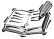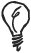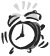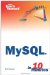# Numeric Operators

In Lesson 4, "Filtering and Sorting Data," you saw how the comparison operators in MySQL were used to restrict the number of rows returned by a query.

Numeric operators are used in a similar waytwo values appear on either side of a symbol or a sequence of symbols. This expression equates to a new value in the SQL statement.## Operators and Operands

In the expression a + b, the + symbol is the operator and a and b are known as operands.

#### Using Arithmetic Operators

An expression that uses a numeric operator can be used in a SQL statement anywhere that you could otherwise put a numeric value. You can also use a numeric operator to modify retrieved data from a table, as long as it is numeric data.

You can actually perform a query in MySQL without supplying a table name. This is useful only when you have an expression as a selected value, but it can be used to show the result of an expression on fixed values.

Addition in MySQL is performed using the + operator, and subtraction using the - operator. The following query shows an expression using each of these operators:

` mysql> SELECT 15 + 28, 94 - 55; +-------- +---------+ | 15 + 28 | 94 - 55 | +---------+---------+ |      43 |      39 | +---------+---------+ 1 row in set (0.00 sec) `

A query with no table list returns exactly one row but can contain multiple columns. In this example, the two columns returned contain the results of the two expressions in the SELECT statement.

The other basic arithmetic operators in MySQL are * and /, for multiplication and division, respectively. The / operator in MySQL returns a decimal numberit does not truncate the result or round it to the nearest whole number.

` mysql> SELECT 6 * 8, 72 / 9, 2 / 3; +-------+--------+-------+ | 6 * 8 | 72 / 9 | 2 / 3 | +-------+--------+-------+ |    48 |   8.00 |  0.67 | +-------+--------+-------+ 1 row in set (0.00 sec) `## Precedence

The *, /, DIV, and MOD operators have a higher precedence than + and -. You can use parentheses where necessary to alter the order in which an expression is evaluated.

The DIV operator can be used to perform division where only a whole number is returned and any remainder is simply discarded. The modulo operator is used to find the remainder after division and is performed using MOD or the % symbol.

The following query divides 20 by 3; the result is 6 with a remainder of 2.

` mysql> SELECT 20 DIV 3, 20 % 3; +----------+--------+ | 20 DIV 3 | 20 % 3 | +----------+--------+ |        6 |      2 | +----------+--------+ 1 row in set (0.00 sec) `

#### Calculated Columns

In the following example, the query retrieves price data from the products table. The third column contains an expression that applies a fixed discount to the price of each item.

` mysql> SELECT code, price, price - 1.50     -> FROM products; +------+-------+--------------+ | code | price | price - 1.50 | +------+-------+--------------+ | MINI |  5.99 |         4.49 | | MIDI |  9.99 |         8.49 | | MAXI | 15.99 |        14.49 | +------+-------+--------------+ 3 rows in set (0.00 sec) `

The expression is evaluated for each row in the result set, and the calculated value is returned in its own column.

Notice that the heading of the calculated column shows the expression in full. If the column value is calculated as the result of a long expression, this could make the query output much too wide. If you want to give this column its own name, you can use the AS keyword to specify a column alias.

In the following example, sales tax of 10% is calculated by multiplying the database price by 0.1 using the multiplication operator, *. The calculated column is given an alias tax.

` mysql> SELECT code, price, price * 0.1 AS tax     -> FROM products; +------+-------+------+ | code | price | tax  | +------+-------+------+ | MINI |  5.99 | 0.60 | | MIDI |  9.99 | 1.00 | | MAXI | 15.99 | 1.60 | +------+-------+------+ 3 rows in set (0.02 sec) `## Column Aliases

In addition to making the column headings more readable in the mysql program, aliases are required when you want to refer to a column by name in your application. By giving a column an alias in your query, you also make it identifiable to a programming language that can interface with MySQL. You will learn how various programming languages can fetch data from a MySQL database in Part VI, "Interfacing with MySQL."

#### NULL Values

If a database column does not contain any data, this is represented in MySQL as NULL. A NULL is no valueit is not the same as zero.

If you perform any kind of arithmetic in which one of the arguments is NULL, the result will also be NULL, as shown in the following example:

` mysql> SELECT 10 + NULL, 10 / NULL, 10 % NULL; +-----------+-----------+-----------+ | 10 + NULL | 10 / NULL | 10 % NULL | +-----------+-----------+-----------+ |      NULL |      NULL |      NULL | +-----------+-----------+-----------+ 1 row in set (0.00 sec) `## Checking for NULL

If you want to check for NULL in a query, use IS NULL or IS NOT NULL. Comparing column = NULL does not work.Sams Teach Yourself MySQL in 10 Minutes
ISBN: 0672328631
EAN: 2147483647
Year: 2006
Pages: 165
Authors: Chris Newman

Similar book on Amazon

flylib.com © 2008-2017.
If you may any questions please contact us: flylib@qtcs.net